# Special relativity and Lorentz Transformation Exercise

• R3ap3r42

#### R3ap3r42

Misplaced Homework Thread -- Moved from the Technical Forums
Summary:: Special relativity and Lorentz Transformations - I got this problem from a first-semester course at university. I have been struggling for a few days and decided to get some help.

A rocket sets out from x = x' = 0 at t = t' = 0 and moves with speed u in the negative x'-direction, as measured in S'.
After 1 year has elapsed in S', the rocket turns around.
According to observers in S, the rocket turns around at x = −1/5 light-year and it returns to the origin in S' at x = 4 light-years.

i) Calculate v/c.

ii) Calculate u/c.

iii) At what time in S does the rocket return to the origin in S'?

Please show your working. We can help you with your homework but we're not going to do it for you. You also need to state what are S and v, since you haven't defined those.

Hi there,
This is not exactly homework, it is just a hobby for me.
I am a software engineer who enjoys doing physics for fun...

I got stuck pretty much at the start. I tried a few things but they all seem to get to a dead-end.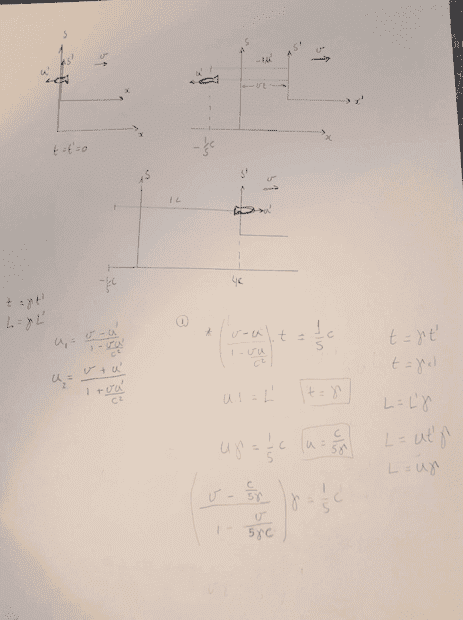S is the stationary frame and S' is the one moving at v in relation to S.

Last edited:
I got stuck pretty much at the start. I tried a few things but they all seem to get to a dead-end.
Welcome to PF. All schoolwork-type questions go in the Homework Help forums, per the PF rules.

I tried to enhance your picture, but no luck so far. Could you please take a better picture or scan your work and try again? Thank you.

Done. Let me know if it is still not clear.

Summary:: Special relativity and Lorentz Transformations - I got this problem from a first-semester course at university. I have been struggling for a few days and decided to get some help.

A rocket sets out from x = x' = 0 at t = t' = 0 and moves with speed u in the negative x'-direction, as measured in S'.
After 1 year has elapsed in S', the rocket turns around.
According to observers in S, the rocket turns around at x = −1/5 light-year and it returns to the origin in S' at x = 4 light-years.

i) Calculate v/c.

ii) Calculate u/c.

iii) At what time in S does the rocket return to the origin in S'?
I suggest doing this problem using non-relativistic mechanics first. Then, if you are comfortable doing that, try the relativistic version.

Are you sure about those S and S primes? Which one is the rest frame of the rocket?

I suggest doing this problem using non-relativistic mechanics first. Then, if you are comfortable doing that, try the relativistic version.
PS I had a look at this. It's quite tricky. I did it non-relativistically first to get a grip on what was happening kinematically. That's important, I think, to really understand what's going on before you start.

The main solution used the Lorentz Transformations. The trick is choosing which transformation to use first. If you write them all down, then one is simpler than the others.

I also calculated the relativistic speeds of the rocket in the S frame and double-checked the answer that way.

Are you sure about those S and S primes? Which way me is the rest frame of the rocket?
##u## in the problem is the speed of the rocket (both ways) in the S' frame. Perhaps it should have been ##u'##. The speed of the rocket in the S frame is different before and after the turnaround.

Are you sure about those S and S primes? Which one is the rest frame of the rocket?
Yes, this is an exact extract from Manchester University First Semester Physics for the 2019 exam.

Yes, this is an exact extract from Manchester University First Semester Physics for the 2019 exam.
As above, try writing down all four Lorentz Transformations. I.e. we have two events: ##E_1## is the turnaround and ##E_2## is when the rocket gets back to the ##S'## spatial origin. We have the LT and inverse LT of both of these. I wrote them all down first.

Not the easiest problem I've ever seen.

I'm still struggling to read that.

There's a fairly simple recipe for solving problems of this nature.
1. Do not use length contraction or time dilation. They are special case formulae and easy to misapply where they are inappropriate.
2. Write down the coordinates of any events of interest in either frame - typically either ##(x,t)## or ##(x',t')##, but actually any two of the four will do.
3. Apply the Lorentz transforms to get the coordinates in the frame you don't have them.
4. Decide which frame you want to be working into get the answer requested. Use coordinate differences in that frame
Looking at the problem statement, it looks like you don't actually have all of the coordinates you'll need - a lot of them are implied by things like the fact that ##x=4## when the rocket reaches ##x'=0##. So the first step, I think, is to think about writing down the coordinates of events you do know and seeing how you can go about filling in the blanks. A table like this might help:$$\begin{array}{|l|c|c|c|c|} \hline \mathrm{Event}&x / \mathrm{ly}&t/ \mathrm{years}&x'/ \mathrm{ly}&t' / \mathrm{years}\\ \hline \mathrm{Rocket\ leaves}&0&0&0&0\\ \mathrm{Rocket\ turns\ around}&-1/5&&&1\\ \mathrm{Rocket\ returns}&4&&0&\\ \hline \end{array}$$

Last edited:
•berkeman
##u## in the problem is the speed of the rocket (both ways) in the S' frame. Perhaps it should have been ##u'##. The speed of the rocket in the S frame is different before and after the turnaround.

The rocket moves at -u and then u in the S' frame we need to the expression for the speed of the rocket in the S frame is trivial w1 = (v-u)/(1-vu/c^2) and w2 = (v+u)/(1+vu/c^2)
I think we need a system of equation to get rid of u first so we can get v/c
I am getting stuck on which equations to use. My attempts resulted in the wrong answer multiple times.

For the S frame, the rocket traveled at speed w1 on the first leg of the journey for a time t. So the first equation I assumed was:

w1*t = 1/5*c

but t = γ*t' and t'=1 so

w1*γ = 1/5*c

This gives me the wrong result for v/c.

The rocket moves at -u and then u in the S' frame we need to the expression for the speed of the rocket in the S frame is trivial w1 = (v-u)/(1-vu/c^2) and w2 = (v+u)/(1+vu/c^2)
I think we need a system of equation to get rid of u first so we can get v/c
I am getting stuck on which equations to use. My attempts resulted in the wrong answer multiple times.
It should be possible this way, but it looked messy, so I used the LT's instead.
For the S frame, the rocket traveled at speed w1 on the first leg of the journey for a time t. So the first equation I assumed was:

w1*t = 1/5*c

but t = γ*t' and t'=1 so

w1*γ = 1/5*c

This gives me the wrong result for v/c.
This is wrong. Time dilation is a special case where events are colocated in the relevant frame. You can't apply time dilation across events that have different spatial coordinates in the relevant frame, You must use the full LT.

Can you give me a hint?

a) 0.894c
b) 0.984c
c) 4.47 years

Here's a hint and something that doesn't seem to be in textbooks. If you generate the quantity ##\gamma v##, which happens quite often. Note that:
$$\gamma v = w \ \Rightarrow \ \frac{v^2}{1 - v^2} = w^2 \ \Rightarrow \ v^2 =\frac{w^2}{1 + w^2}$$$$\Rightarrow \ v = \frac{w}{\sqrt{1 + w^2}} \ \text{and} \ \gamma = \sqrt{1 + w^2}$$That's how you extract ##v## and ##\gamma## from ##\gamma v##. Note that I've used ##c=1##.

Can you give me a hint?

a) 0.894c
b) 0.984c
c) 4.47 years
That's what I get. The hint is to write down the inverse Lorentz transformations:
$$E_1 = (t_1, x_1) = \dots, \ E_2 = (t_2, x_2) = \dots$$
And, you'll need the two events in the S' frame:
$$E'_1 = (t'_1, x'_1) = \dots, \ E'_2 = (t'_2, x'_2) = \dots$$Where these can be specified as much as possible.

I may be missing something... that is what I see from the post...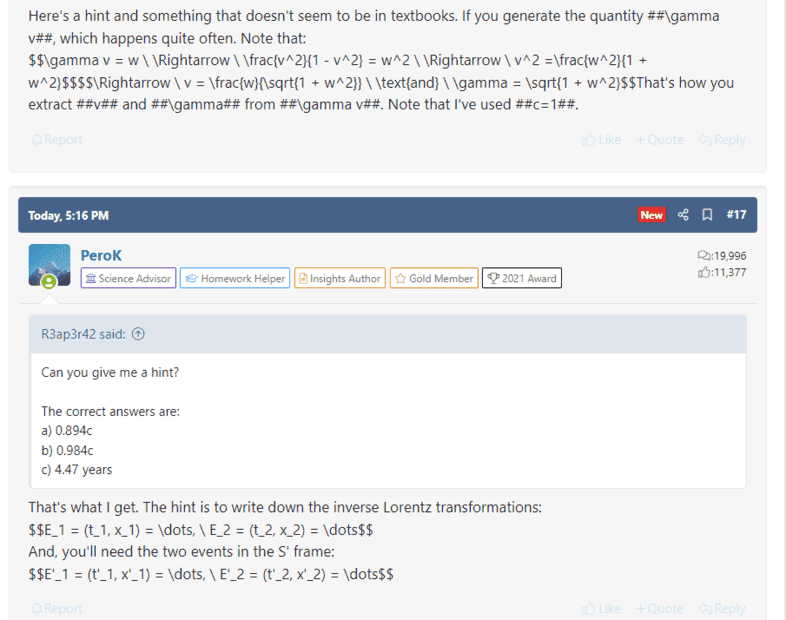Do I need any extension on my browser?

Nevermind I was using Brave it works on Chrome.

•PeroK
Nevermind I was using Brave it works on Chrome.
MathJax is a Javascript package, and quite a computationally hefty one, so it's lazily loaded. Sometimes it's a bit too lazy and you need to refresh the page to get it to realize it needs to do something.

I'm still struggling to read that.

There's a fairly simple recipe for solving problems of this nature.
1. Do not use length contraction or time dilation. They are special case formulae and easy to misapply where they are inappropriate.
2. Write down the coordinates of any events of interest in either frame - typically either ##(x,t)## or ##(x',t')##, but actually any two of the four will do.
3. Apply the Lorentz transforms to get the coordinates in the frame you don't have them.
4. Decide which frame you want to be working into get the answer requested. Use coordinate differences in that frame
Looking at the problem statement, it looks like you don't actually have all of the coordinates you'll need - a lot of them are implied by things like the fact that ##x=4## when the rocket reaches ##x'=0##. So the first step, I think, is to think about writing down the coordinates of events you do know and seeing how you can go about filling in the blanks. A table like this might help:$$\begin{array}{|l|c|c|c|c|} \hline \mathrm{Event}&x / \mathrm{ly}&t/ \mathrm{years}&x'/ \mathrm{ly}&t' / \mathrm{years}\\ \hline \mathrm{Rocket\ leaves}&0&0&0&0\\ \mathrm{Rocket\ turns\ around}&-1/5&&&1\\ \mathrm{Rocket\ returns}&4&&0&\\ \hline \end{array}$$
That is my understanding but I fail to generate the needed equations from that.

That is my understanding but I fail to generate the needed equations from that.
You need to use the Lorentz Transformations.

That is my understanding but I fail to generate the needed equations from that.
You should be able to complete the table in terms of ##v##. You'll then have to think about how you could get some expressions for ##u## (hint: think about what observers using frame S say about the rocket's motion).

Are you sure about those S and S primes? Which one is the rest frame of the rocket?
Neither S nor S' is the rest frame of the rocket.

I am still struggling so I will post what I have so far...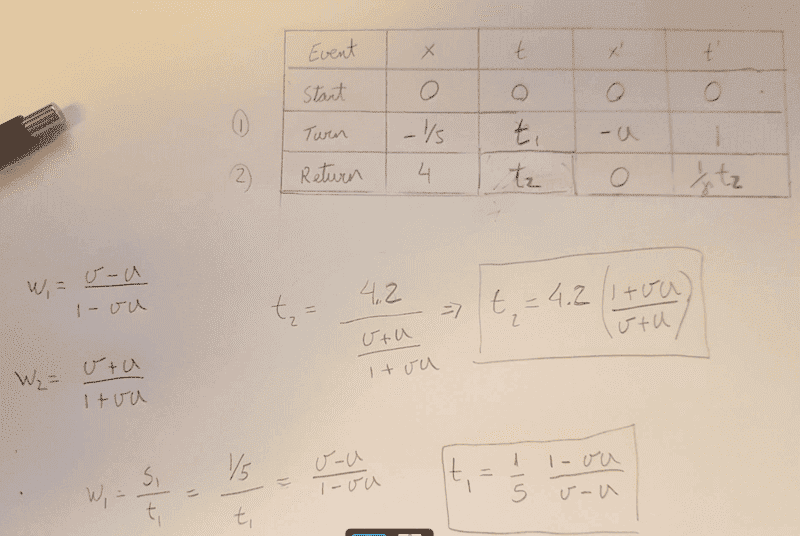Are these correct?

Neither S nor S' is the rest frame of the rocket.
The question is as posted in the exam. Word for word.

I am still struggling so I will post what I have so far...

View attachment 295780

Are these correct?
You should be able to write down ##t'_2##.

I don't think you'll get a solution very easily using velocity transformations. Lorentz transformations are the way to go. I might have said that already.

A table like this might help:$$\begin{array}{|l|c|c|c|c|} \hline \mathrm{Event}&x / \mathrm{ly}&t/ \mathrm{years}&x'/ \mathrm{ly}&t' / \mathrm{years}\\ \hline \mathrm{Rocket\ leaves}&0&0&0&0\\ \mathrm{Rocket\ turns\ around}&-1/5&&&1\\ \mathrm{Rocket\ returns}&4&&0&\\ \hline \end{array}$$
I find spacetime diagrams really helpful as well to keep things straight.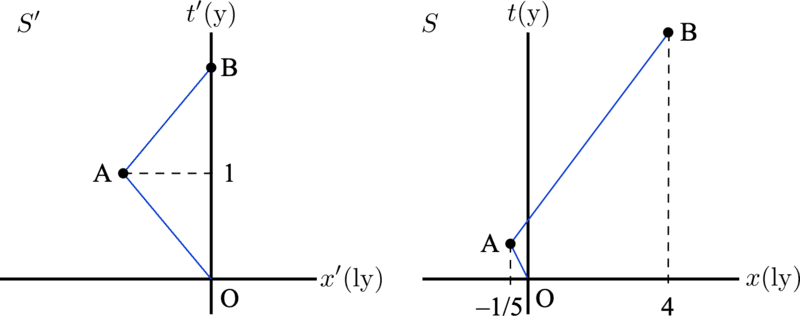event O = rocket leaves
event A = rocket turns around
event B = rocket returns

As @PeroK noted, it should be pretty clear what ##t'_B## (PeroK's ##t'_2##) is equal to from the diagram on the left.

•BvU and R3ap3r42
Spacetime diagrams are an excellent tool.

@R3ap3r42 - the point with the Lorentz transforms is that you can use them to complete each row of the table in terms of ##v## because in each case you know two things, ##x## and either ##x'## or ##t'##. The Lorentz transforms are two equations, and there are two things you want to know..

Only at that point do you bring in the fact you've already observed, that ##x'## at the turn around is ##u\times 1##. You'll also need the value of ##t'## at the return, which you should be able to deduce). Then you can solve for ##v## then ##u##.

•R3ap3r42
OK, this helps a little more.
I was happy for a minute there until I got again to something wrong...
Where did I mess it up this time?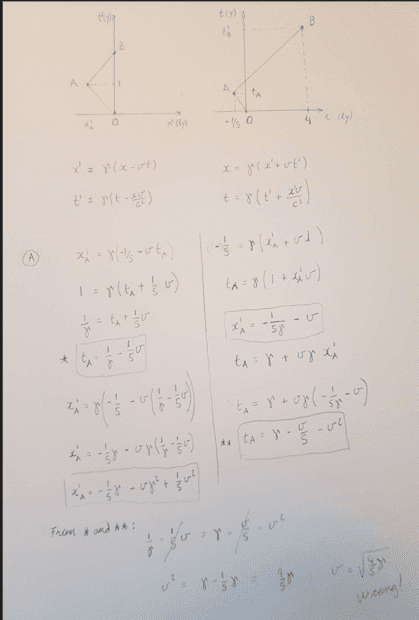Did you get that ##t'_B =2## years? Note that that is also true in classical mechanics.

I did suggest writing down all of the LTs and finding the simplest one. That was:$$x_B = \gamma(0 +vt'_B)$$That gives you a value for the quantity ##\gamma v##. I explained in a previous post how to deal with that.

•R3ap3r42
I finally got it.
a) and c) were straightforward and match the expected result.
For b) I got 2 different values depending on if I use O to A or A to B to get u.
Is this correct? If so the question should have specified which direction right?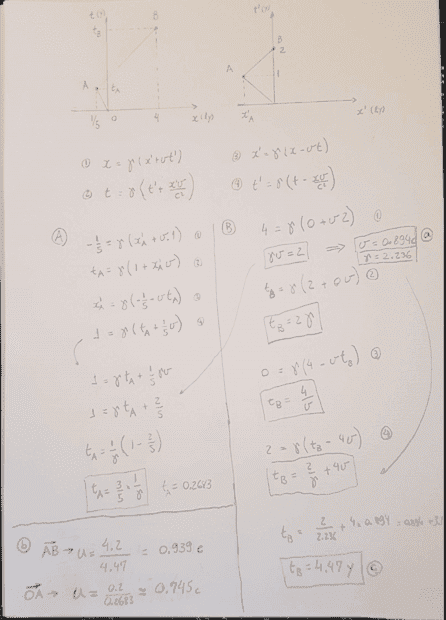My sincere thanks to @PeroK, @Ibix, @vela etc. I really appreciate the tough-love. I can honestly say I have learned loads just with this one question.

•PeroK and BvU
Here's my minimialist solution (natural units):
$$x_B = \gamma(0 + vt'_B) = 2\gamma v \ \Rightarrow \ \gamma = \sqrt 5, v = \frac 2 5 \sqrt 5$$$$t_B = \gamma(t'_B) = 2\gamma = 2\sqrt 5$$$$x_A = \gamma(x'_A + vt'_A) \ \Rightarrow x'_A = -\frac{11}{25}\sqrt 5$$$$x'_A = -ut'_A \ \Rightarrow \ u = \frac{11}{25}\sqrt 5$$To double check, we calculate the speeds of the rocket in the S frame:
$$u_A = \frac{-u + v}{1-uv} = -\frac{1}{3}\sqrt 5 \ \Rightarrow \ t_A = \frac{x_A}{u_A} = \frac{3}{25}\sqrt 5$$$$u_B = \frac{u + v}{1+uv} = \frac{21}{47}\sqrt 5$$And we can confirm that:$$x_B = u_At_A + u_B(t_B-t_A) = 4$$

Last edited:
•Ibix and R3ap3r42
For b) I got 2 different values depending on if I use O to A or A to B to get u.
Is this correct? If so the question should have specified which direction right?
You should have gotten the same speed for both directions. Remember that ##u## is the speed of the rocket as seen by an observer at rest in ##S'##. The speed you calculated using OA, for instance, is the speed of the rocket an observer at rest in ##S## would see.

•R3ap3r42
Yes, I understand that now. I got the values described by @PeroK, which are the velocities S sees the rocket coming and going.
The question asked u in the S' frame so only one value for both legs of the trip.
Thanks a lot for all the help.